# Deep Level Transient Spectroscopy Using HF2LI Lock-in Amplifier

May 23, 2015 by James Wei

Co-author and main contributor: Dr. Antonio Braga

## Introduction

A very small concentration of lattice point defects, or simply defects, such as vacancies, impurities, dislocations, grain boundaries or cavities, are responsible for creating many different properties in semiconductors. Defects play a crucial (either beneficial or detrimental) role in determining the suitability in terms of performance and reliability of a specific material to be used in a semiconductor device. The identification (i.e. energy of deep levels in the bandgap) and control (i.e. quantification) of defects is among the most important tasks in materials and electronic device engineering.

Deep Level Transient Spectroscopy (DLTS) is an experimental electrical characterization technique that yields information about the temperature dependence of active trapping centers (formed by point defects) that exist in the depletion region of semiconductor devices or single junctions. This technique is normally applied to a junction with abrupt separation of carrier dopants, such as asymmetrical pn junctions (i.e. one side of the junction heavily doped and the other side lightly doped) or Schottky junctions. A number of different DLTS measurements have been developed. The most popular ones are the conventional Rate Window technique, Fourier transform and the Laplace transform methods [Tin 2012].

This blog refers to the conventional DLTS [Lang 1974] in which the junction capacitance changes (i.e. transient) are measured when electron trapping centers within the junction are filled and discharged through a voltage pulse. The rate at which electrons escape the traps (i.e. the emission rate) is highly temperature dependent. Therefore, the activation energy of the trapping level from the nearest energy band edge and the trap capture cross-section can be determined by obtaining the emission rate's temperature dependence. The main purpose of the blog is to illustrate the DLTS setup with the HF2LI Lock-in Amplifier and to perform data extraction through emission rate measurement.

## Experimental Setup

HF2LI is the core of the DLTS experimental apparatus. It simultaneously generates the DUT probing signal which consists of overlapping the external voltage pulse with a high frequency sinusoidal modulation signal, and the out-of-phase sinusoidal signal used for capacitance compensation. HF2LI can also be used to measure and record the capacitance transient at a very high sampling rate.

In addition to HF2LI, the setup requires two additional pieces of equipment: a cryostat to change the sample temperature, and a pulse generator. In this case, an Agilent 33500B suffices as pulse generator which only needs to generate a continuous pulse train which alternates the DUT in reverse and zero bias. This pulse train is added to the modulation signal through the ADD port. The pulse train does not have to be fast. In fact, it has to be slow enough for the transient to reach steady-state. The old Booton capacitance meter which was popular for DLTS sets a fixed modulation frequency at 1 MHz. With HF2LI, one can set the modulation to any arbitrary frequency. One has to be aware that if the frequency is too low, than the signal-to-noise ratio will be poorer because the impedance of a capacitor increases as frequency decreases (i.e. measurement current gets smaller).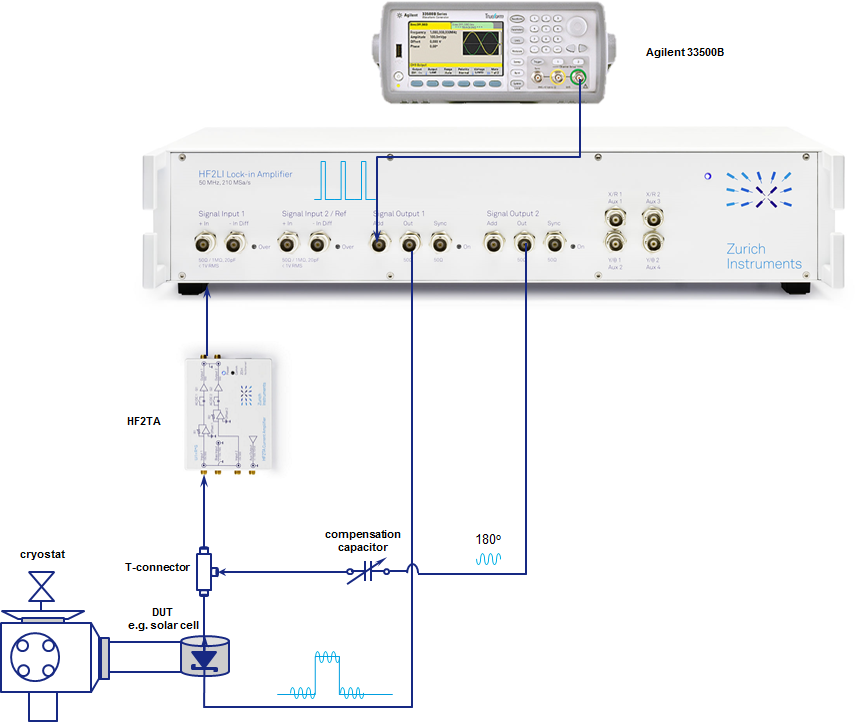The capacitance transient is in fact more like a current transient. This transient happens when the DUT is forced from reverse bias into forward bias. The HF2TA transimpedance amplifier measures the current passing through the sample at a given modulation frequency. The demodulated data represents a direct measurement of the capacitance/current transient.

Given there will be continuous transients due to the reverse-bias pulse train, HF2LI can continuously record as many capacitance transients as one would like at a desired demodulator sampling rate using the poll command for a defined recording time. An example of Matlab script used to setup the HF2LI and to record the transient is given here. An averaged transient produced from the pulse train can be easily combined and averaged into one single transient with a Matlab script for better signal-to-noise ratio. The more pulses generated in a single measurement the better the effect of noise averaging will be naturally. A 'clean' transient is essential for applying the Window Rate Technique which will be detailed later on.

## Capacitance Compensation

The idea of enhancing capacitance measurement sensitivity using HF2LI can be found in this blog. The DUT's capacitance normally consists of a constant part and a variable part which varies with the bias. If the capacitance variation is a small fraction of the overall constant capacitance value, the capacitance compensation with a reference capacitor should be made (as described in the aforementioned blog) in order to increase capacitance change detection sensitivity. This compensation procedure is not mandatory but can sometimes help to improve measurement quality.

## Emission Rate Determination with the Rate Window Technique

The rate window technique is the original conventional DLTS technique and remains the fundamental one. This method is based on determining the capacitance difference at two different times, t1 and t2, recorded during capacitance transient being stimulated by a proper bias voltage pulse. For simplicity, let us assume a single trap energy level. Then, the transient capacitance C(t) can be written in a general way as:

C(t)=C0[1-N·exp(-ent)]

where C0 is the capacitance junction under reverse bias condition, N accounts for the concentration of electron traps and dopant concentration, t is the acquisition time, and en is the emission rate.

The DLTS signal (S) is defined as the difference between two capacitance measured during the transient in two different times, namely t1 and t2. Therefore, S will be given by the following equation:

S = Ct1 – Ct2 = C0N[exp(-ent1)+ exp(-ent2)]

where Cti is the capacitance measured at the time ti.

Furthermore, the capacitance decay transient changes as a function of the temperature. The time constant at low temperature is longer because the low emission rate of the trapped electrons. As the temperature of the sample increases, the time constant becomes shorter due to a faster rate of emission of the trapped electrons due to the increased thermal energy. If we select a window by choosing a starting point t1 with an arbitrary ratio t2/t1, we can then get a DLTS curve by calculating S for all the transients from different temperatures. To illustrate this clearly, an example of such a curve (right) derived from a series of simulated transient curves (left) is shown below. Each colored point on the S curve represents the value obtained from a transient window at different temperatures. The most important characteristic on this S curve is its minimum point.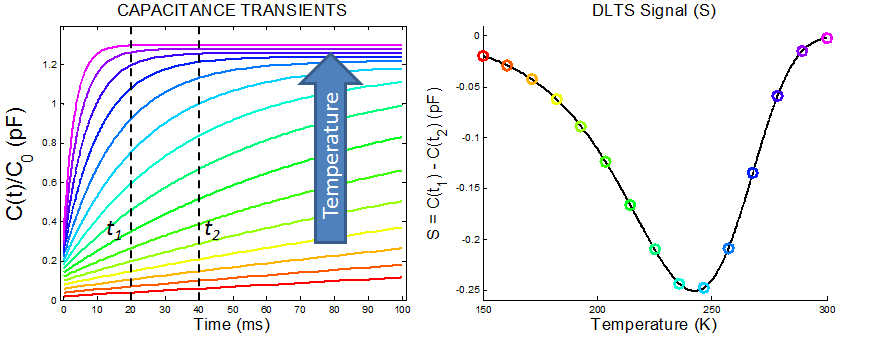Simulated curves for illustration purposes

To obtain multiple S curves, one can simply 'sweep' the window. This is done by keeping the same t2/t1 ratio and simply move the starting t1 point. And this can be done using a script in post-processing. In reality, the S curves will not look as symmetrical as the one simulated above because the temperature range chosen might be too small. Depending on the location of the windowing chosen, the S curve might be quite asymmetrical and will not always produce a minimum point within the measurement temperature range.

It is nevertheless to obtain as many curves with a minimum as possible for the subsequent Arrhenius data analysis. There are several ways to get more such minima. One obvious way is to record transients over a temperature range as wide as possible. But this means more measurement time. A better way would be to do some smart data post processing. On top of sweeping t1, one can also create a larger number of windows by choosing a smaller t2/t1 ratio. The plots below are from experimental data gathered from a series of measured transients over temperature and post-processed with a Matlab script. More S curves with visible minima within the measured temperature range are created by post-possessing. The obvious minimum points are marked with a red cross. In fact, there would not have been enough minima without this post-processing step for a meaningful data extraction.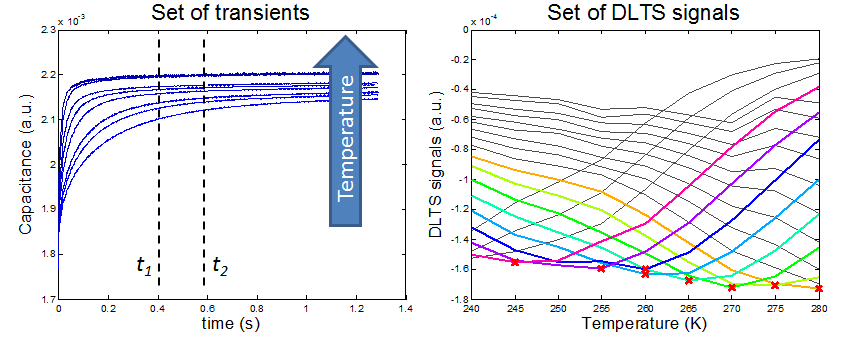Actual measurement data

## Data Fitting and Analysis

For the previous curves with a minimum derived from measured transients, it can be seen that each red cross can be linked to a certain temperature. Let us call it Tmin. At these Tmin, the emission rate en is a function of the time delay parameters t1 and t2 with the equation:

en=ln(t2/t1)/(t2-t1)

Now that we have a data set consists of [Tmin en], the next step is to draw an Arrhenius plot. An Arrhenius plot is a graph of logarithmic kinetic constants plotted against the inverse temperature. In this case, the kinetic constants ln(en/Tmin2) are plotted against 1/kBTmin. From a theoretical approach, there exists a linear relation between the previous parameters:

ln(en/Tmin2) = -ΔE/kBTmin + ln(σn) + ln(16πme*kB2/(gh3))

where, kB is the Boltzmann constant, σn is the capture cross-section of the traps and ΔE is the activation energy of the traps (with respect to the conduction band edge), me* is the electron effective mass, g is the degeneracy factor, and h is the Planck constant [Tin 2012]. The last term is a constant term related to the process.

It must be said that the red crosses marked in the DLTS curves are close to but not exactly the actual minima due to the coarseness of the temperature intervals chosen. It is therefore recommended to do some fitting so the more realistic Tmin can be estimated. The graph below is obtained through data fitting of the actual DLTS curves with a Matlab script. In this example, the DLTS signal curves appeared to have an asymmetrical shape. Different fitting algorithms including double Gaussian, double Lorentzian and 4th and 6th order polynomial were tested. In the end, polynomial fit was chosen to achieve the most suitable fit. It can be seen that fitting is important since there is an offset between the measured minima and the fitted minima. The fitting can also help to smooth out the scattering of various data points.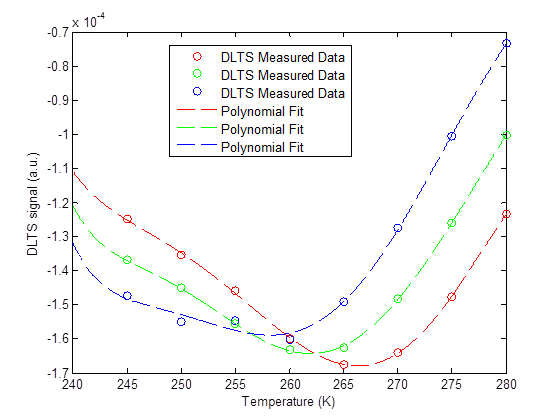From the previous equation as well as the new fitted data set, an Arrhenius plot can be generated as shown below. The slope of the straight interpolating line gives the activation energy of the electron trap ΔE which is estimated be about 400 meV. The y-intercept of this straight line gives the value ln(σn) + ln(16πme*kB2/(gh3)) with ln(16πme*kB2/(gh3)) being a constant . The capture cross-section σn of the electron trap can be calculated when the constant term is calculated with the process parameters.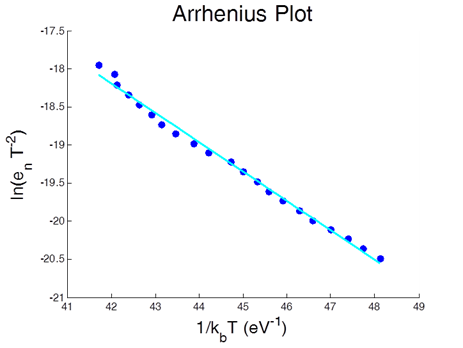## Conclusion

In this blog, a description of the conventional DLTS measurement using the Zurich Instruments HF2LI Lock-in Amplifier was given. A step-by-step explanation was also given for extracting the electron trap activation energy ΔE as well as the trap capture cross section parameter σn. One key point is that HF2LI Lock-in Amplifier is able to demodulate and record transient behaviour at very high speed and resolution which generate ample data points for post processing interpolation and fitting. This eliminates the need to make measurements at many small temperature steps thus can save a lot of characterization time. If you have any comments or questions regarding performing DLTS measurement, we will happy to hear them.

## Acknowledgement

We would like to thank Dr. Arouna Darga from Laboratoire de Génie Électrique at Supélec, France, for providing Zurich Instruments the measurement setup with the cryostat as well as the measurement sample for the DLTS measurement.

## References

Tin, C.-C. 2012. Deep Level Transient Spectroscopy. Characterization of Materials. 1–14. Lang, D. V. 1974. Deep-level transient spectroscopy: A new method to characterize traps in semiconductors. J. Appl. Phys. 45:3023–3032. Zurich Instrument 2017 Laplace Deep Level Transient Spectroscopy using the MFIA